# The PANEL Procedure

## Getting Started: PANEL Procedure

The following statements use the cost function data from Greene (1990) to estimate the variance components model. The variable PRODUCTION is the log of output in millions of kilowatt-hours, and COST is the log of cost in millions of dollars. See Greene (1990) for details.

data greene;
input firm year production cost @@;
datalines;
1 1955   5.36598   1.14867  1 1960   6.03787   1.45185
1 1965   6.37673   1.52257  1 1970   6.93245   1.76627
2 1955   6.54535   1.35041  2 1960   6.69827   1.71109
2 1965   7.40245   2.09519  2 1970   7.82644   2.39480
3 1955   8.07153   2.94628  3 1960   8.47679   3.25967

... more lines ...



You decide to fit the following model to the data: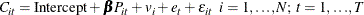where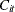and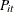represent the cost and production, and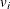,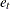and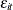are the cross-sectional, time series, and error variance components.

If you assume that the time and cross-sectional effects are random, you are left with four possible estimators for the variance components. You choose Fuller-Battese.

The following statements fit this model.

proc sort data=greene;
by firm year;
run;

proc panel data=greene;
model cost = production / rantwo vcomp = fb;
id firm year;
run;


The PANEL procedure output is shown in Figure 20.1. A model description is printed first, which reports the estimation method used and the number of cross sections and time periods. The variance components estimates are printed next. Finally, the table of regression parameter estimates shows the estimates, standard errors, and t tests.

Figure 20.1: The Variance Components Estimates

The PANEL Procedure
Fuller and Battese Variance Components (RanTwo)

Dependent Variable: cost

Model Description
Estimation Method RanTwo
Number of Cross Sections 6
Time Series Length 4

Fit Statistics
SSE 0.3481 DFE 22
MSE 0.0158 Root MSE 0.1258
R-Square 0.8136

Variance Component Estimates
Variance Component for Cross Sections 0.046907
Variance Component for Time Series 0.00906
Variance Component for Error 0.008749

Hausman Test for Random Effects
DF m Value Pr > m
1 26.46 <.0001

Parameter Estimates
Variable DF Estimate Standard
Error
t Value Pr > |t|
Intercept 1 -2.99992 0.6478 -4.63 0.0001
production 1 0.746596 0.0762 9.80 <.0001